CATEGORIES:

Asymptotes of a Hyperbola

Definition. The asymptote of a curve is a straight line approached by the curve line at infinity.

An oblique asymptote is determined by an equation of the form an oblique

y=kx+b.

To find the slope, we suppose that b=0; then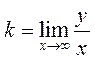;.

We find b from the equation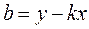.

Passing to the limit, we obtainThus, the hyperbola has two asymptotes, passing through the origin: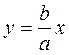;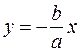.

First, we construct a rectangle with sides 2а along the х-axis and 2b along the у-axis. We draw the diagonals in this rectangle and extend them; they are the asymptotes of the hyperbola.y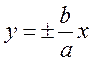2a 2b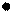F1 М1(–а;0) 0 М2(а;0) F2 x

From the vertices M1(–a;0) and M2(a;0), we draw the branches of the hyperbola approaching the asymptotes.

The directrices of a hyperbola. By definition, . By analogy with an ellipse, we find the difference of squares,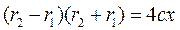.

Date: 2015-01-02; view: 892

 <== previous page | next page ==> Tangents to an Ellipse | As a result, we obtain
doclecture.net - lectures - 2014-2022 year. Copyright infringement or personal data (0.001 sec.)